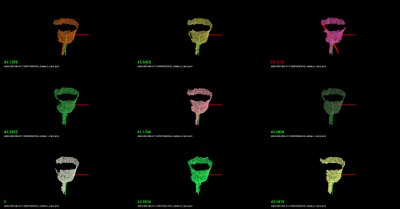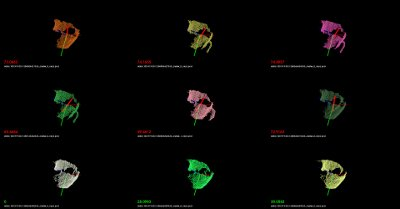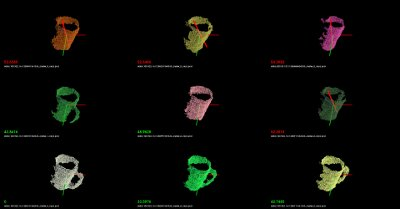# Cluster Recognition and 6DOF Pose Estimation using VFH descriptors

As previously described in Estimating VFH signatures for a set of points, Viewpoint Feature Histograms (VFH) are powerful meta-local descriptors, created for the purpose of recognition and pose estimation for clusters of points. We here refer to a cluster as a collection of 3D points, most of the time representing a particular object or part of a scene, obtained through some segmentation or detection mechanisms (please see Euclidean Cluster Extraction for an example).

Our goal here is not to provide an ultimate recognition tool, but rather a mechanism for obtaining candidates that could potentially be the cluster/object that is searched for, together with its 6DOF pose in space. With this in mind, we will be formulating the recognition problem as a nearest neighbor estimation problem. So given a set of training data, we will use efficient nearest neighbor search structures such as kd-trees and return a set of potential candidates with sorted distances to the query object, rather than an absolute “this is the object that we were searching for” kind of response. The reader can imagine that such a system becomes much more useful as we can explicitly reason about failures (false positives, or true negatives).

For the purpose of this tutorial, the application example could be formulated as follows:

• Training stage:

• given a scene with 1 object that is easily separable as a cluster;

• use a ground-truth system to obtain its pose (see the discussion below);

• rotate around the object or rotate the object with respect to the camera, and compute a VFH descriptor for each view;

• store the views, and build a kd-tree representation.

• Testing stage:

• given a scene with objects that can be separated as individual clusters, first extract the clusters;

• for each cluster, compute a VFH descriptor from the current camera position;

• use the VFH descriptor to search for candidates in the trained kd-tree.

We hope the above makes sense. Basically we’re first going to create the set of objects that we try to later on recognize, and then we will use that to obtain valid candidates for objects in the scene.

A good example of a ground-truth system could be a simple rotating pan-tilt unit such as the one in the figure below. Placing an object on the unit, and moving it with some increments in both horizontal and vertical, can result in a perfect ground-truth system for small objects. A cheaper solution could be to use a marker-based system (e.g., checkerboard) and rotate the camera/table manually.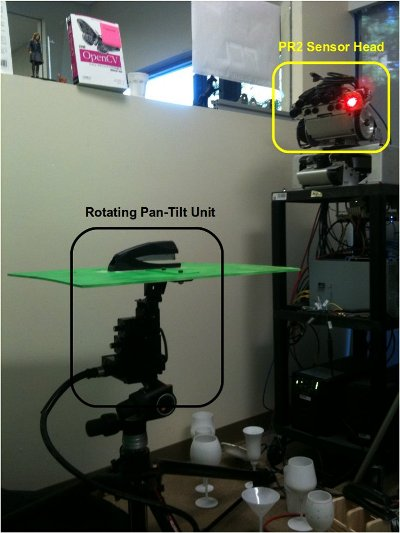Our Kd-Tree implementation of choice for the purpose of this tutorial is of course, FLANN.

# Training

We begin the training by assuming that the objects are already separated as individual clusters (see Euclidean Cluster Extraction), as shown in the figure below: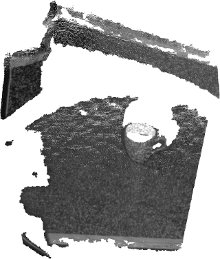Since we’re only trying to cover the explicit training/testing of VFH signatures in this tutorial, we provide a set of datasets already collected at: vfh_recognition_tutorial_data.tbz. The data is a subset of the objects presented in the figure below (left), and look like the point clouds on the right. We used the pan-tilt table shown above to acquire the data.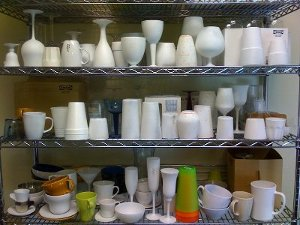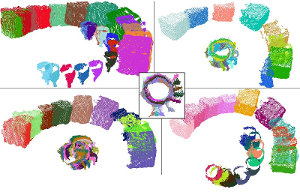Next, copy and paste the following code into your editor and save it as `build_tree.cpp`.

```  1#include <pcl/point_types.h>
2#include <pcl/point_cloud.h>
3#include <pcl/console/print.h>
4#include <pcl/io/pcd_io.h>
5#include <boost/filesystem.hpp>
6#include <flann/flann.h>
7#include <flann/io/hdf5.h>
8#include <fstream>
9
10
11typedef std::pair<std::string, std::vector<float> > vfh_model;
12
13/** \brief Loads an n-D histogram file as a VFH signature
14  * \param path the input file name
15  * \param vfh the resultant VFH model
16  */
17bool
18loadHist (const boost::filesystem::path &path, vfh_model &vfh)
19{
20  int vfh_idx;
21  // Load the file as a PCD
22  try
23  {
24    pcl::PCLPointCloud2 cloud;
25    int version;
26    Eigen::Vector4f origin;
27    Eigen::Quaternionf orientation;
29    int type; unsigned int idx;
31
32    vfh_idx = pcl::getFieldIndex (cloud, "vfh");
33    if (vfh_idx == -1)
34      return (false);
35    if ((int)cloud.width * cloud.height != 1)
36      return (false);
37  }
38  catch (const pcl::InvalidConversionException&)
39  {
40    return (false);
41  }
42
43  // Treat the VFH signature as a single Point Cloud
44  pcl::PointCloud <pcl::VFHSignature308> point;
46  vfh.second.resize (308);
47
48  std::vector <pcl::PCLPointField> fields;
49  pcl::getFieldIndex<pcl::VFHSignature308> ("vfh", fields);
50
51  for (std::size_t i = 0; i < fields[vfh_idx].count; ++i)
52  {
53    vfh.second[i] = point.histogram[i];
54  }
55  vfh.first = path.string ();
56  return (true);
57}
58
59/** \brief Load a set of VFH features that will act as the model (training data)
60  * \param argc the number of arguments (pass from main ())
61  * \param argv the actual command line arguments (pass from main ())
62  * \param extension the file extension containing the VFH features
63  * \param models the resultant vector of histogram models
64  */
65void
66loadFeatureModels (const boost::filesystem::path &base_dir, const std::string &extension,
67                   std::vector<vfh_model> &models)
68{
69  if (!boost::filesystem::exists (base_dir) && !boost::filesystem::is_directory (base_dir))
70    return;
71
72  for (boost::filesystem::directory_iterator it (base_dir); it != boost::filesystem::directory_iterator (); ++it)
73  {
74    if (boost::filesystem::is_directory (it->status ()))
75    {
76      std::stringstream ss;
77      ss << it->path ();
79      loadFeatureModels (it->path (), extension, models);
80    }
81    if (boost::filesystem::is_regular_file (it->status ()) && boost::filesystem::extension (it->path ()) == extension)
82    {
83      vfh_model m;
84      if (loadHist (base_dir / it->path ().filename (), m))
85        models.push_back (m);
86    }
87  }
88}
89
90int
91main (int argc, char** argv)
92{
93  if (argc < 2)
94  {
95    PCL_ERROR ("Need at least two parameters! Syntax is: %s [model_directory] [options]\n", argv);
96    return (-1);
97  }
98
99  std::string extension (".pcd");
100  transform (extension.begin (), extension.end (), extension.begin (), (int(*)(int))tolower);
101
102  std::string kdtree_idx_file_name = "kdtree.idx";
103  std::string training_data_h5_file_name = "training_data.h5";
104  std::string training_data_list_file_name = "training_data.list";
105
106  std::vector<vfh_model> models;
107
108  // Load the model histograms
110  pcl::console::print_highlight ("Loaded %d VFH models. Creating training data %s/%s.\n",
111      (int)models.size (), training_data_h5_file_name.c_str (), training_data_list_file_name.c_str ());
112
113  // Convert data into FLANN format
114  flann::Matrix<float> data (new float[models.size () * models.second.size ()], models.size (), models.second.size ());
115
116  for (std::size_t i = 0; i < data.rows; ++i)
117    for (std::size_t j = 0; j < data.cols; ++j)
118      data[i][j] = models[i].second[j];
119
120  // Save data to disk (list of models)
121  flann::save_to_file (data, training_data_h5_file_name, "training_data");
122  std::ofstream fs;
123  fs.open (training_data_list_file_name.c_str ());
124  for (std::size_t i = 0; i < models.size (); ++i)
125    fs << models[i].first << "\n";
126  fs.close ();
127
128  // Build the tree index and save it to disk
129  pcl::console::print_error ("Building the kdtree index (%s) for %d elements...\n", kdtree_idx_file_name.c_str (), (int)data.rows);
130  flann::Index<flann::ChiSquareDistance<float> > index (data, flann::LinearIndexParams ());
131  //flann::Index<flann::ChiSquareDistance<float> > index (data, flann::KDTreeIndexParams (4));
132  index.buildIndex ();
133  index.save (kdtree_idx_file_name);
134  delete[] data.ptr ();
135
136  return (0);
137}
```

In the following paragraphs we will explain what the above code does (or should do). We’ll begin with the `main` function.

We begin by loading a set of feature models from a directory given as the first command line argument (see details for running the example below). The `loadFeatureModels` method does nothing but recursively traverse a set of directories and subdirectories, and loads in all .PCD files it finds. In `loadFeatureModels`, we call `loadHist`, which will attempt to open each PCD file found, read its header, and check whether it contains a VFH signature or not. Together with the VFH signature we also store the PCD file name into a `vfh_model` pair.

Once all VFH features have been loaded, we convert them to FLANN format, using:

```  // Convert data into FLANN format
flann::Matrix<float> data (new float[models.size () * models.second.size ()], models.size (), models.second.size ());

for (std::size_t i = 0; i < data.rows; ++i)
for (std::size_t j = 0; j < data.cols; ++j)
data[i][j] = models[i].second[j];
```

Since we’re lazy, and we want to use this data (and not reload it again by crawling the directory structure in the testing phase), we dump the data to disk:

```  // Save data to disk (list of models)
flann::save_to_file (data, training_data_h5_file_name, "training_data");
std::ofstream fs;
fs.open (training_data_list_file_name.c_str ());
for (std::size_t i = 0; i < models.size (); ++i)
fs << models[i].first << "\n";
fs.close ();
```

Finally, we create the KdTree, and save its structure to disk:

```  pcl::console::print_error ("Building the kdtree index (%s) for %d elements...\n", kdtree_idx_file_name.c_str (), (int)data.rows);
flann::Index<flann::ChiSquareDistance<float> > index (data, flann::LinearIndexParams ());
//flann::Index<flann::ChiSquareDistance<float> > index (data, flann::KDTreeIndexParams (4));
index.buildIndex ();
index.save (kdtree_idx_file_name);
```

Here we will use a `LinearIndex`, which does a brute-force search using a Chi-Square distance metric (see [VFH] for more information). For building a proper kd-tree, comment line 1 and uncomment line 2 in the code snippet above. The most important difference between a LinearIndex and a KDTreeIndex in FLANN is that the KDTree will be much faster, while producing approximate nearest neighbor results, rather than absolute.

So, we’re done with training. To summarize:

1. we crawled a directory structure, looked at all the .PCD files we found, tested them whether they are VFH signatures and loaded them in memory;

2. we converted the data into FLANN format and dumped it to disk;

3. we built a kd-tree structure and dumped it to disk.

# Testing

In the testing phase, we will illustrate how the system works by randomly loading one of the files used in the training phase (feel free to supply your own file here!), and checking the results of the tree.

Begin by copying and pasting the following code into your editor and save it as `nearest_neighbors.cpp`.

```  1#include <pcl/point_types.h>
2#include <pcl/point_cloud.h>
3#include <pcl/common/common.h>
4#include <pcl/common/centroid.h> // for compute3DCentroid
5#include <pcl/visualization/pcl_visualizer.h>
6#include <pcl/console/parse.h>
7#include <pcl/console/print.h>
8#include <pcl/io/pcd_io.h>
9#include <iostream>
10#include <limits>
11#include <flann/flann.h>
12#include <flann/io/hdf5.h>
13#include <boost/filesystem.hpp>
14#include <boost/algorithm/string/replace.hpp> // for replace_last
15typedef std::pair<std::string, std::vector<float> > vfh_model;
16
17/** \brief Loads an n-D histogram file as a VFH signature
18  * \param path the input file name
19  * \param vfh the resultant VFH model
20  */
21bool
22loadHist (const boost::filesystem::path &path, vfh_model &vfh)
23{
24  int vfh_idx;
25  // Load the file as a PCD
26  try
27  {
28    pcl::PCLPointCloud2 cloud;
29    int version;
30    Eigen::Vector4f origin;
31    Eigen::Quaternionf orientation;
33    int type; unsigned int idx;
35
36    vfh_idx = pcl::getFieldIndex (cloud, "vfh");
37    if (vfh_idx == -1)
38      return (false);
39    if ((int)cloud.width * cloud.height != 1)
40      return (false);
41  }
42  catch (const pcl::InvalidConversionException&)
43  {
44    return (false);
45  }
46
47  // Treat the VFH signature as a single Point Cloud
48  pcl::PointCloud <pcl::VFHSignature308> point;
50  vfh.second.resize (308);
51
52  std::vector <pcl::PCLPointField> fields;
53  pcl::getFieldIndex<pcl::VFHSignature308> ("vfh", fields);
54
55  for (std::size_t i = 0; i < fields[vfh_idx].count; ++i)
56  {
57    vfh.second[i] = point.histogram[i];
58  }
59  vfh.first = path.string ();
60  return (true);
61}
62
63
64/** \brief Search for the closest k neighbors
65  * \param index the tree
66  * \param model the query model
67  * \param k the number of neighbors to search for
68  * \param indices the resultant neighbor indices
69  * \param distances the resultant neighbor distances
70  */
71inline void
72nearestKSearch (flann::Index<flann::ChiSquareDistance<float> > &index, const vfh_model &model,
73                int k, flann::Matrix<int> &indices, flann::Matrix<float> &distances)
74{
75  // Query point
76  flann::Matrix<float> p = flann::Matrix<float>(new float[model.second.size ()], 1, model.second.size ());
77  memcpy (&p.ptr (), &model.second, p.cols * p.rows * sizeof (float));
78
79  indices = flann::Matrix<int>(new int[k], 1, k);
80  distances = flann::Matrix<float>(new float[k], 1, k);
81  index.knnSearch (p, indices, distances, k, flann::SearchParams (512));
82  delete[] p.ptr ();
83}
84
85/** \brief Load the list of file model names from an ASCII file
86  * \param models the resultant list of model name
87  * \param filename the input file name
88  */
89bool
90loadFileList (std::vector<vfh_model> &models, const std::string &filename)
91{
92  std::ifstream fs;
93  fs.open (filename.c_str ());
94  if (!fs.is_open () || fs.fail ())
95    return (false);
96
97  std::string line;
98  while (!fs.eof ())
99  {
100    std::getline (fs, line);
101    if (line.empty ())
102      continue;
103    vfh_model m;
104    m.first = line;
105    models.push_back (m);
106  }
107  fs.close ();
108  return (true);
109}
110
111int
112main (int argc, char** argv)
113{
114  int k = 6;
115
116  double thresh = std::numeric_limits<double>::max();     // No threshold, disabled by default
117
118  if (argc < 2)
119  {
120    pcl::console::print_error
121      ("Need at least three parameters! Syntax is: %s <query_vfh_model.pcd> [options] {kdtree.idx} {training_data.h5} {training_data.list}\n", argv);
122    pcl::console::print_info ("    where [options] are:  -k      = number of nearest neighbors to search for in the tree (default: ");
123    pcl::console::print_value ("%d", k); pcl::console::print_info (")\n");
124    pcl::console::print_info ("                          -thresh = maximum distance threshold for a model to be considered VALID (default: ");
125    pcl::console::print_value ("%f", thresh); pcl::console::print_info (")\n\n");
126    return (-1);
127  }
128
129  std::string extension (".pcd");
130  transform (extension.begin (), extension.end (), extension.begin (), (int(*)(int))tolower);
131
132  // Load the test histogram
133  std::vector<int> pcd_indices = pcl::console::parse_file_extension_argument (argc, argv, ".pcd");
134  vfh_model histogram;
135  if (!loadHist (argv[pcd_indices.at (0)], histogram))
136  {
137    pcl::console::print_error ("Cannot load test file %s\n", argv[pcd_indices.at (0)]);
138    return (-1);
139  }
140
141  pcl::console::parse_argument (argc, argv, "-thresh", thresh);
142  // Search for the k closest matches
143  pcl::console::parse_argument (argc, argv, "-k", k);
144  pcl::console::print_highlight ("Using "); pcl::console::print_value ("%d", k); pcl::console::print_info (" nearest neighbors.\n");
145
146  std::string kdtree_idx_file_name = "kdtree.idx";
147  std::string training_data_h5_file_name = "training_data.h5";
148  std::string training_data_list_file_name = "training_data.list";
149
150  std::vector<vfh_model> models;
151  flann::Matrix<int> k_indices;
152  flann::Matrix<float> k_distances;
153  flann::Matrix<float> data;
154  // Check if the data has already been saved to disk
155  if (!boost::filesystem::exists ("training_data.h5") || !boost::filesystem::exists ("training_data.list"))
156  {
157    pcl::console::print_error ("Could not find training data models files %s and %s!\n",
158        training_data_h5_file_name.c_str (), training_data_list_file_name.c_str ());
159    return (-1);
160  }
161  else
162  {
165    pcl::console::print_highlight ("Training data found. Loaded %d VFH models from %s/%s.\n",
166        (int)data.rows, training_data_h5_file_name.c_str (), training_data_list_file_name.c_str ());
167  }
168
169  // Check if the tree index has already been saved to disk
170  if (!boost::filesystem::exists (kdtree_idx_file_name))
171  {
172    pcl::console::print_error ("Could not find kd-tree index in file %s!", kdtree_idx_file_name.c_str ());
173    return (-1);
174  }
175  else
176  {
177    flann::Index<flann::ChiSquareDistance<float> > index (data, flann::SavedIndexParams ("kdtree.idx"));
178    index.buildIndex ();
179    nearestKSearch (index, histogram, k, k_indices, k_distances);
180  }
181
182  // Output the results on screen
183  pcl::console::print_highlight ("The closest %d neighbors for %s are:\n", k, argv[pcd_indices]);
184  for (int i = 0; i < k; ++i)
185    pcl::console::print_info ("    %d - %s (%d) with a distance of: %f\n",
186        i, models.at (k_indices[i]).first.c_str (), k_indices[i], k_distances[i]);
187
189  pcl::visualization::PCLVisualizer p (argc, argv, "VFH Cluster Classifier");
190  int y_s = (int)std::floor (sqrt ((double)k));
191  int x_s = y_s + (int)std::ceil ((k / (double)y_s) - y_s);
192  double x_step = (double)(1 / (double)x_s);
193  double y_step = (double)(1 / (double)y_s);
194  pcl::console::print_highlight ("Preparing to load ");
195  pcl::console::print_value ("%d", k);
196  pcl::console::print_info (" files (");
197  pcl::console::print_value ("%d", x_s);
198  pcl::console::print_info ("x");
199  pcl::console::print_value ("%d", y_s);
200  pcl::console::print_info (" / ");
201  pcl::console::print_value ("%f", x_step);
202  pcl::console::print_info ("x");
203  pcl::console::print_value ("%f", y_step);
204  pcl::console::print_info (")\n");
205
206  int viewport = 0, l = 0, m = 0;
207  for (int i = 0; i < k; ++i)
208  {
209    std::string cloud_name = models.at (k_indices[i]).first;
210    boost::replace_last (cloud_name, "_vfh", "");
211
212    p.createViewPort (l * x_step, m * y_step, (l + 1) * x_step, (m + 1) * y_step, viewport);
213    l++;
214    if (l >= x_s)
215    {
216      l = 0;
217      m++;
218    }
219
220    pcl::PCLPointCloud2 cloud;
222    if (pcl::io::loadPCDFile (cloud_name, cloud) == -1)
223      break;
224
225    // Convert from blob to PointCloud
226    pcl::PointCloud<pcl::PointXYZ> cloud_xyz;
227    pcl::fromPCLPointCloud2 (cloud, cloud_xyz);
228
229    if (cloud_xyz.size () == 0)
230      break;
231
232    pcl::console::print_info ("[done, ");
233    pcl::console::print_value ("%zu", static_cast<std::size_t>(cloud_xyz.size ()));
234    pcl::console::print_info (" points]\n");
235    pcl::console::print_info ("Available dimensions: ");
236    pcl::console::print_value ("%s\n", pcl::getFieldsList (cloud).c_str ());
237
238    // Demean the cloud
239    Eigen::Vector4f centroid;
240    pcl::compute3DCentroid (cloud_xyz, centroid);
241    pcl::PointCloud<pcl::PointXYZ>::Ptr cloud_xyz_demean (new pcl::PointCloud<pcl::PointXYZ>);
242    pcl::demeanPointCloud<pcl::PointXYZ> (cloud_xyz, centroid, *cloud_xyz_demean);
245
246    // Check if the model found is within our inlier tolerance
247    std::stringstream ss;
248    ss << k_distances[i];
249    if (k_distances[i] > thresh)
250    {
251      p.addText (ss.str (), 20, 30, 1, 0, 0, ss.str (), viewport);  // display the text with red
252
253      // Create a red line
254      pcl::PointXYZ min_p, max_p;
255      pcl::getMinMax3D (*cloud_xyz_demean, min_p, max_p);
256      std::stringstream line_name;
257      line_name << "line_" << i;
258      p.addLine (min_p, max_p, 1, 0, 0, line_name.str (), viewport);
259      p.setShapeRenderingProperties (pcl::visualization::PCL_VISUALIZER_LINE_WIDTH, 5, line_name.str (), viewport);
260    }
261    else
262      p.addText (ss.str (), 20, 30, 0, 1, 0, ss.str (), viewport);
263
264    // Increase the font size for the score*
265    p.setShapeRenderingProperties (pcl::visualization::PCL_VISUALIZER_FONT_SIZE, 18, ss.str (), viewport);
266
267    // Add the cluster name
268    p.addText (cloud_name, 20, 10, cloud_name, viewport);
269  }
270  // Add coordinate systems to all viewports
272
273  p.spin ();
274  return (0);
275}
```

The above code snippet is slightly larger, because we also included some visualization routines and some other “eye candy” stuff.

In lines:

```  // Load the test histogram
std::vector<int> pcd_indices = pcl::console::parse_file_extension_argument (argc, argv, ".pcd");
vfh_model histogram;
{
pcl::console::print_error ("Cannot load test file %s\n", argv[pcd_indices.at (0)]);
return (-1);
}

pcl::console::parse_argument (argc, argv, "-thresh", thresh);
// Search for the k closest matches
pcl::console::parse_argument (argc, argv, "-k", k);
```

we load the first given user histogram (and ignore the rest). Then we proceed at checking two command line parameters, namely `-k` which will define how many nearest neighbors to check and display on screen, and `-thresh` which defines a maximum distance metric after which we will start displaying red lines (i.e., crossing) over the k models found on screen (eye candy!).

In lines:

```  {
```

we load the training data from disk, together with the list of file names that we previously stored in `build_tree.cpp`. Then, we read the kd-tree and rebuild the index:

```  {
flann::Index<flann::ChiSquareDistance<float> > index (data, flann::SavedIndexParams ("kdtree.idx"));
```

Here we need to make sure that we use the exact distance metric (`ChiSquareDistance` in this case), as the one that we used while creating the tree. The most important part of the code comes here:

```    index.buildIndex ();
```

Inside `nearestKSearch`, we first convert the query point to FLANN format:

```  // Query point
flann::Matrix<float> p = flann::Matrix<float>(new float[model.second.size ()], 1, model.second.size ());
```

Followed by obtaining the resultant nearest neighbor indices and distances for the query in:

```  memcpy (&p.ptr (), &model.second, p.cols * p.rows * sizeof (float));

indices = flann::Matrix<int>(new int[k], 1, k);
distances = flann::Matrix<float>(new float[k], 1, k);
```

Lines:

```    flann::Index<flann::ChiSquareDistance<float> > index (data, flann::SavedIndexParams ("kdtree.idx"));
index.buildIndex ();
nearestKSearch (index, histogram, k, k_indices, k_distances);
}

// Output the results on screen
pcl::console::print_highlight ("The closest %d neighbors for %s are:\n", k, argv[pcd_indices]);
for (int i = 0; i < k; ++i)
pcl::console::print_info ("    %d - %s (%d) with a distance of: %f\n",
i, models.at (k_indices[i]).first.c_str (), k_indices[i], k_distances[i]);

pcl::visualization::PCLVisualizer p (argc, argv, "VFH Cluster Classifier");
int y_s = (int)std::floor (sqrt ((double)k));
int x_s = y_s + (int)std::ceil ((k / (double)y_s) - y_s);
```

create a `PCLVisualizer` object, and sets up a set of different viewports (e.g., splits the screen into different chunks), which will be enabled in:

```
```

Using the file names representing the models that we previously obtained in `loadFileList`, we proceed at loading the model file names using:

```    pcl::PCLPointCloud2 cloud;
if (pcl::io::loadPCDFile (cloud_name, cloud) == -1)
break;

// Convert from blob to PointCloud
pcl::PointCloud<pcl::PointXYZ> cloud_xyz;
```

For visualization purposes, we demean the point cloud by computing its centroid and then subtracting it:

```    // Demean the cloud
Eigen::Vector4f centroid;
pcl::compute3DCentroid (cloud_xyz, centroid);
pcl::PointCloud<pcl::PointXYZ>::Ptr cloud_xyz_demean (new pcl::PointCloud<pcl::PointXYZ>);
pcl::demeanPointCloud<pcl::PointXYZ> (cloud_xyz, centroid, *cloud_xyz_demean);
```

Finally we check if the distance obtained by `nearestKSearch` is larger than the user given threshold, and if it is, we display a red line over the cloud that is being rendered in the viewport:

```      // Create a red line
pcl::PointXYZ min_p, max_p;
pcl::getMinMax3D (*cloud_xyz_demean, min_p, max_p);
std::stringstream line_name;
line_name << "line_" << i;
p.addLine (min_p, max_p, 1, 0, 0, line_name.str (), viewport);
```

# Compiling and running the code

Create a new `CMakeLists.txt` file, and put the following content into it

``` 1cmake_minimum_required(VERSION 3.5 FATAL_ERROR)
2
3project(vfh_cluster_classifier)
4
5find_package(PCL 1.2 REQUIRED)
6include_directories(\${PCL_INCLUDE_DIRS})
9
10find_package(HDF5 REQUIRED)
11find_package(FLANN REQUIRED)
12
13include_directories(SYSTEM
14  \${HDF5_INCLUDE_DIR}
15)
16
19                                 FLANN::FLANN \${HDF5_LIBRARIES})
20
```

Note

If you are running this tutorial on Windows, you have to install (HDF5 1.8.7 Shared Library). If CMake is not able to find HDF5, you can manually supply the include directory in HDF5_INCLUDE_DIR variable and the full path of hdf5dll.lib in HDF5_hdf5_LIBRARY variable. Make sure that the needed dlls are in the same folder as the executables.

The above assumes that your two source files (`build_tree.cpp` and `nearest_neighbors.cpp`) are stored into the src/ subdirectory.

Then, make sure that the datasets you downloaded (vfh_recognition_tutorial_data.tbz) are unpacked in this directory, thus creating a data/ subdirectory.

After you have made the executable, you can run them like so:

```\$ ./build/build_tree data/
```

You should see the following output on screen:

```> Loading data/001.324.25 (0 models loaded so far).
> Loaded 195 VFH models. Creating training data training_data.h5/training_data.list.
Building the kdtree index (kdtree.idx) for 195 elements...
```

The above crawled the data/ subdirectory, and created a kd-tree with 195 entries. To run the nearest neighbor testing example, you have two options:

1. Either run the following command manually, and select one of the datasets that we provided as a testing sample, like this:

```./build/nearest_neighbors -k 16 -thresh 50 data/000.580.67/1258730231333_cluster_0_nxyz_vfh.pcd
```
2. Or, if you are on a linux system, you can place the following on a bash script file (e.g., `test.sh`):

```#!/bin/bash

# Example directory containing _vfh.pcd files
DATA=data

# Inlier distance threshold
thresh=50

# Get the closest K nearest neighbors
k=16

for i in `find \$DATA -type d -name "*"`
do
echo \$i
for j in `find \$i -type f \( -iname "*cluster*_vfh.pcd" \) | sort -R`
do
echo \$j
./build/nearest_neighbors -k \$k -thresh \$thresh \$j -cam "0.403137,0.868471/0,0,0/-0.0932051,-0.201608,-0.518939/-0.00471487,-0.931831,0.362863/1464,764/6,72"
done
done
```

and run the script like this:

```bash test.sh
```

You should see recognition examples like the ones shown below: